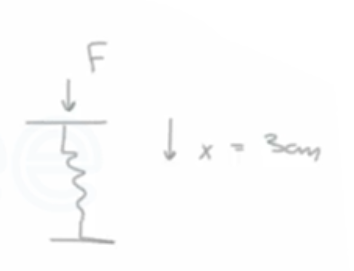Need Help?

Subscribe to Thermodynamics

###### \${selected_topic_name}
• Notes

$\begin{array}{l}{\text { Determine the energy required to accelerate an } 800-\mathrm{kg}} \\ {\text { car from rest to } 100 \mathrm{km} / \mathrm{hon} \text { a level road }}\end{array}$

$m=800 \mathrm{kg}$

$V_{1}=0$

$V_{2}=100 \mathrm{km} / \mathrm{h} \longrightarrow$$\frac{1000}{3600}=2.778 \mathrm{m} / \mathrm{s}$

$w_{a}=\frac{1}{2} m\left(v_{2}^{2}-v_{1}^{2}\right)$

$=\frac{1}{2}(800)\left(2.778^{2}\right)*$$\frac{1kg}{1000 kg m^2/s^2}$

$={309 \mathrm{KJ}}$

$\begin{array}{l}{\text { How much work, in } \mathrm{kJ} \text { , can a spring whose spring constant }} \\ {\text { is } 3 \mathrm{kN} / \mathrm{cm} \text { produce after it has been compressed } 3 \mathrm{cm}} \\ {\text { from its unloaded length? }}\end{array}$$W=\int_{1}^{2} F d s=\int_{1}^{2} k x d x$

$=k \int_{1}^{2} x d x$

$=\frac{k}{2}\left(x_{2}^{2}-x_{1}^{2}\right)$

$=\frac{300}{2}\left(0.03^{2}-0^{2}\right)=0.135 \mathrm{kN} \cdot \mathrm{m}$

$\frac{1kJ}{1{k N \cdot m}} \longrightarrow W=0.135 \mathrm{k}{J}$